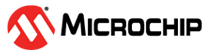• All
• Products
• Documents
• Applications Notes

# Fixed Point Math Library for PIC24 and dsPIC

 The Fixed Point Math Library simplifies writing fixed point algorithms, supporting both Q15 and Q16 data formats. Using the simple, C callable functions contained in the library complex fixed point mathematical operations can be easily executed. The library is built into the MPLAB C Compiler and is part of every install. Description The Fixed Point Math Library contains functions for manipulating Q15 and Q16 integers. The 16-bit Language Tools document details the operation of the Q15 and Q16 formats and explains each function in detail. • Compatible with the Microchip PIC24and dsPIC families • Over 30 math functions for working with Q15 or Q16 data • Detailed explanation of Q formatted data • Detailed description of each function • Highly optimized functions for fast execution Devices Supported All processors in the PIC24and dsPIC DSC family Getting Started • Download and install MPLAB and the free trial version of the C compiler • See Chapter 5 of the 16-bit Language Tools Library for complete documentation of the Fixed Point Math Library • Start writing Q formatted math code! Common Fixed Point Math Functions Function Description _Q1Xabs* Absolute value of a Q15/Q16 value _Q1Xsin* Sin of a Q15/Q16 number _Q1Xcos* Cos of a Q15/Q16 number _Q1Xtan* Tan of a Q15/Q16 number _Q1Xasin* Arc sin of a Q15/Q16 value _Q1Xacos* Arc cos of a Q15/Q16 value _Q1Xatan* Arc tan of a Q15/Q16 value _Q1Xadd* Sums two Q15/Q16 values, taking care of overflow and underflow _Q1Xsub* Subtracts two Q15/Q16 values, taking care of overflow and underflow _Q1Xlog* Log of a Q15/Q16 number _Q1Xatoi* Converts ASCII number to a Q15/Q16 number _Q1Xftoi* Converts a single precision floating point number to a Q1X value _itoaQ1X* Converts a Q1X value to an ASCII encoded number _itofQ1X* Converts Q1X value to a floating point value *- function name contains either Q15 or Q16 depending on the variable type A complete list of fixed point math functions is available in the 16-Bit Language Tool Libraries document